December 8, 2023

# Area of Triangles- Definition, Formula and Examples

Area of Triangles: The area of a triangle  or any plane figure is defined as the total space enclosed by  their sides . Unit of measurement for area of any plane figure is taken as square metre () or  square centimetre (

) etc. There are many ways to find the area of a triangle. Heron has  derived the famous formula for the area of a triangle in  terms of its three sides . Trigonometric functions  and determinants are also used to find the area of a triangle .

In this lesson, we will learn the  different methods of finding area of  triangles, along with some examples.

Different formula for finding area of a triangle:

Contents

# Area of Triangles(General Formula)

The basic formula for finding the area of triangle is equal to  half the  product of its base and height. It is applicable to all  types of triangles whether it is scalene, isosceles or equilateral.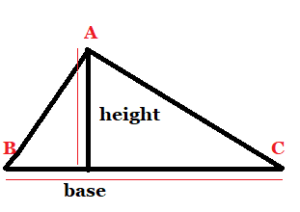(  fig.  1)

 Area of triangle=

When the triangle is right angled ( A triangle in which two sides are perpendicular ) , we can directly apply the formula by using two sides  containing the right angle as base and height. For example, suppose that the sides of a right triangle ABC are 8 cm, 15 cm and 17 cm; we take base as  15 cm and height as 8 cm   (see Fig. 2), then the area of triangle is given by

=

=60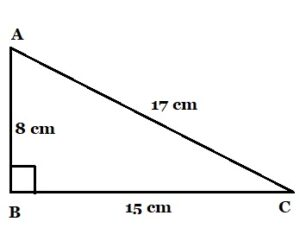(fig. 2)

Note :  We could also take 8 cm as the base and 15 cm as height.

How to find area of triangle with 3 sides ?

## Area of Scalene Triangle( Heron’s Formula)

A triangle whose all sides  are different in length is called scalene triangle. Suppose  we know the  lengths of the sides of a scalene triangle and not  the height.  We can still find its area. Heron has  derived the  formula for the area of a triangle in  terms of its three sides. The formula given by Heron about the area of a triangle, is also known as Heron’s formula. It is stated as:

 Area of a triangle =

where

, and
are the sides of the triangle and s= semi-perimeter i.e. half of the perimeter of the triangle= .

This formula is helpful where it is not possible to find the height of the triangle  easily.

Example :  What is the area of the triangle whose sides are  8 m, 11 m, and 13 m.

Solution :   We have

cm

So,

Therefore , area of the triangle =

=

=

Area of triangle =     .

How to find the area of an equilateral triangle?

### Area of Equilateral triangle

Equilateral  triangle is  a  triangle  in which all the three sides are equal  also all the three  internal angles are equal.  The formula for finding  area of an equilateral triangle  is

 Area of an equilateral triangle =

Derivation for the formula of an equilateral triangle  is given below.

Consider the following equilateral triangle ABC  whose side length is ‘‘ units. Draw the altitude AD (the perpendicular segment from a vertex of a triangle to the opposite side ) .  In an equilateral triangle all its altitude, angle bisector and median from any of its vertices are coincident (they are the same line segment). This can be proved using the concept of congruence of triangles.  since AD is median to BC,   BD=DC=

units.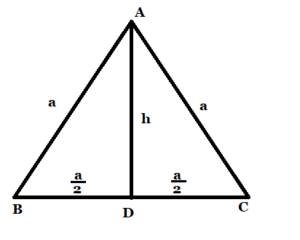Now AD is also the perpendicular to BC, we will use the Pythagorean theorem

(

) to determine its  height AD= h units.  In triangle  ABD,

Area of =

=.

Example : Find the area of an equilateral  triangle whose side is

.

Sol.  Area of an equilateral triangle =

=

=

#### Trigonometric formula for finding area of triangles ( Area of triangle with 2 sides and Included angle)

Consider the

, whose vertex angles are ∠, ∠
, and ∠, and sides are
and
, as shown in the figure below.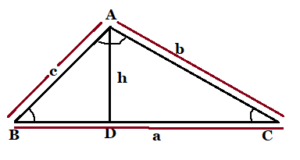Now, if any two sides and the angle between them are given, then the formulas to calculate the area of a triangle is given by:

 ,     and

Since  AD is perpendicular to BC, In

Substituting this value of

in the formula   Area=   , we get
.

This is also known as SAS formula  for the area of a triangle. Similarly we can write the formula in terms of  and

also.

Example:  In ,

, side units, side
= 8 units.

=   =

)

Area

= 12 square units.

##### Area of triangles formula  using determinant

Let  and

be the co Area of a triangle with vertices and
is given by

Note: 1. Since area is a positive quantity , we always take the absolute value of the determinant in finding the area of triangle.

2. If area is given , use both positive and negative values of the determinant for calculation.

3. The area of triangle formed by three collinear  points is zero.

Example:  Find the area of the triangle whose vertices are (3,8), (-4, 2) and (5,1) .

Sol. Area of a triangle with vertices

and is given by

Putting the given values, area of the  triangle  is    =

=

=

sq. units

Area of triangles quizzes

5
Created onTriangle Quizzes

1 / 5

1. The base of a right angled triangle is 8 cm and hypotenuse is 17 cm. Find its area.

2 / 5

2. Find the area of an equilateral triangle whose side is

cm.

3 / 5

3. Find the area of an isosceles triangle whose equal  sides are 5 cm each  and the base is 6 cm.

4 / 5

4. Find the area of an equilateral triangle with side 10 cm.

5 / 5

Find the area of the  given triangle.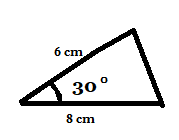The average score is 70%

0%